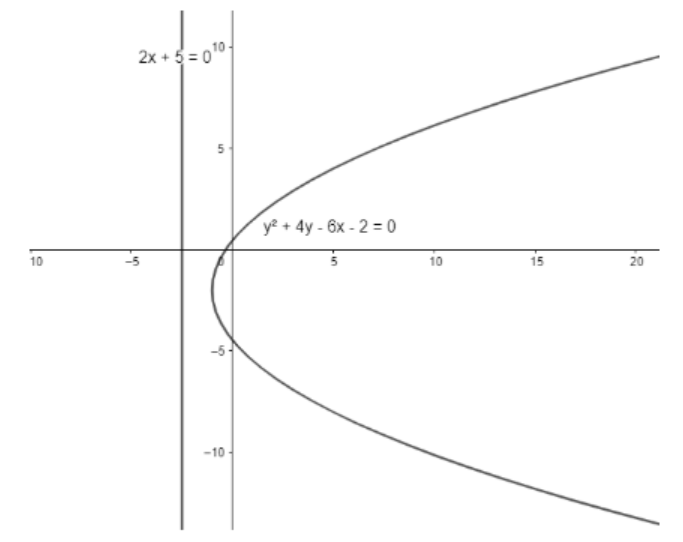QuestionAnswers

# Locus of point of intersection of the perpendicular tangents to the curve ${{y}^{2}}+4y-6x-2=0$ isa) $2x-1=0$b) $2x+3=0$c) $2y+3=0$d) $2x+5=0$Verified
147k+ views
Hint: To find the locus of point of intersection of perpendicular tangents to the parabola, one must know that the point of intersection of perpendicular tangents to any curve is the equation of the director circle of the curve which is the directrix in the case of a parabola.

We have a curve ${{y}^{2}}+4y-6x-2=0$.
We want to find the locus of points from which perpendicular tangents can be drawn to the parabola.
We know that the locus of points of intersection of the perpendicular tangents to a curve is called the director circle.
Rewriting the equation of the curve, we get ${{y}^{2}}+4y=6x+2$
Adding 4 on both sides of the equation, we get ${{y}^{2}}+4y+4=6x+6$
$\Rightarrow {{\left( y+2 \right)}^{2}}=4\times \dfrac{3}{2}\left( x+1 \right)$This is the equation of parabola of a curve. We know for a parabola, the equation of the director circle is the equation of the directrix of parabola.
We know that the directrix is a straight line and not a circle, but it can be assumed to be a circle of infinite radius.
We know the equation of the directrix of parabola of the form ${{\left( y-k \right)}^{2}}=4a\left( x-h \right)$ is $x+a-h=0$.
Comparing with the equation of our parabola, we get $k=-2,a=\dfrac{3}{2},h=-1$
Thus, the equation of director circle (directrix) is $x+\dfrac{3}{2}+1=0$
$\Rightarrow 2x+5=0$, which is the required equation of the director circle.
However, it is not necessary that we will always get the locus of intersection of tangents as a straight line. If the angle between the two tangents changes, the equation of locus of their point of intersection will also change depending upon the angle between the two tangents.
Hence, the correct answer is d) $2x+5=0$.

Note: There’s no need to find the exact equations of tangent and solve for locus. It will lead to a time consuming and longer solution.